• 问答
• 技术
• 实践
• 资源

## 本文目录

21 LV-ViT: 56M参数训练视觉Transformer
(来自 新加坡国立大学冯佳时组 ，字节跳动)
21.1 LV-ViT原理分析

22 通过抑制过度平滑来改进视觉Transformer训练
22.1 原理分析

Transformer 是 Google 的团队在 2017 年提出的一种 NLP 经典模型，现在比较火热的 Bert 也是基于 Transformer。Transformer 模型使用了 Self-Attention 机制，不采用 RNN 的顺序结构，使得模型可以并行化训练，而且能够拥有全局信息。

## 21 LV-ViT: 56M参数训练视觉Transformer

with 56M Parameters on ImageNet

https://arxiv.org/pdf/2104.10858.pdf​arxiv.org

• 21.1 LV-ViT原理分析：

• 1 增加模型的深度： 原始的视觉Transformer是12个block，简单地多加几个block对模型性能没什么改变，这一点已经在DeepViT和CaiT的论文中得到了证实，可参考DeepViT和CaiT这2个模型：

• 2 在模型中引入卷积使之具有inductive bias的能力： 前作已经指出，在视觉Transformer中加入一些卷积操作，能够更容易地提取到low-level的信息 (即edges 和 lines)，这是因为卷积操作具有inductive bias的能力，使得模型在浅层能很好地学习到low-level的信息，这些信息使得输入给 Transformer Block 的patch embedding 更有意义。本文沿用了这一操作，在模型中加入了卷积。同时为了使得卷积的感受野更大，本文使得卷积操作的stride更小，这样一来就能够提供更多的overlapped的信息。在模型中引入卷积这一点已经在CvT，CeiT的论文中得到了证实，具体可以参考下面的链接：

• 3 在残差连接中加入scale factor： 原始Transformer模型的前向过程是这样的：

$$\begin{equation} \begin{split} X \longleftarrow X + \text{SA}(\text{LN}(X)) \ X \longleftarrow X + \text{FF}(\text{LN}(X)) \end{split} \end{equation} \tag{21.1}$$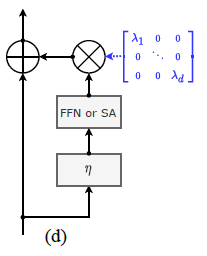• 4 Re-labeling技术： 在训练阶段总是会采用一些数据增强的方法，比较常用的是random crop方法，但是在random crop以后，标签并不总是准确的，因为ImageNet中的许多图像包括多个object，并且具有Ground Truth标签的对象可能不会保留在random crop之后的图像中。这个问题，当random crop的图像越小时就会越严重，因为crop出来的图像越小，可能越不会包含Ground Truth的label信息，如下图3所示。在使用单标签标注时，图像随机剪裁可能包含与真值完全不同的对象，为训练带来噪声甚至不准确的监督信号。所以作者遵循了之前一篇论文：Re-labeling ImageNet: from Single to Multi-Labels, from Global to Localized Labels的解决方法，论文链接如下：

https://arxiv.org/pdf/2101.05022.pdf​arxiv.org

Re-labeling技术的具体做法是使用多标签对 ImageNet 训练集进行重新标注，具体是怎么做的呢？

Re-labeling论文的作者首先在一个巨大的数据集上训练了一个强大的图像分类器模型，这个数据集是JFT-300M或者InstagramNet-1B，并把这个模型在ImageNet上进行fine-tune。 作者认为这个图像分类器模型非常接近人类的预测了。那么因为这个数据集是私有的，并且训练这个模型非常耗费时间和算力 (上百个GPU days)，所以为了后人研究的方便，作者开源了这个模型。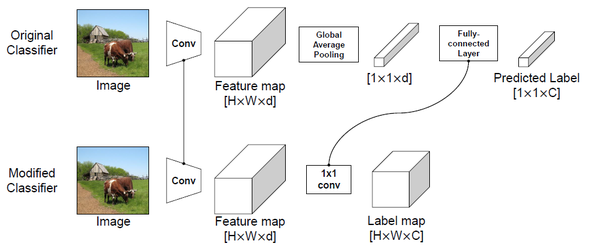Algorithm A1 ReLabel Pseudo-code
for each training iteration do
# Load image data and label maps (assume the minibatch size is 1 for simplicity)
input, label map = get minibatch(dataset)
# Random crop augmentation
[cx,cy,cw,ch] = get crop region(size(input))
input = random crop(input, [cx,cy,cw,ch])
input = resize(input, [224; 224])
# LabelPooling process
target = RoIAlign(label map, coords=[cx,cy,cw,ch], output size=(1; 1))
target = softmax(target)
# Update model
output = model forward(input)
loss = cross entropy loss(output, target)
model update(loss)
end for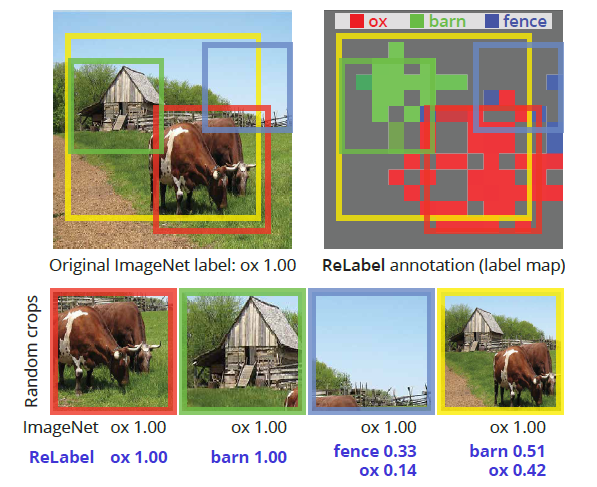• 5 Token labeling：

Token labeling可以用下图4来表述。作者认为模型的输出，即 $[X^{cls},X^1,...,X^N]$ 现在只利用了 $X^{cls}$ ，而剩下的 $N$ 个token其实是可以结合上面的Re-labeling技术加以利用。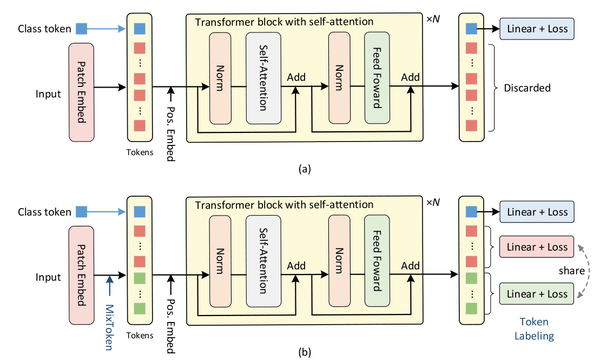$$\begin{equation} L{aux}=\frac{1}{N}\sum{i=1}^N H(X^i,y^i) \end{equation} \tag{21.2}$$

$$\begin{equation} L{total}=H(X^{cls},y^{cls}) + \beta L{aux}, \end{equation} \tag{21.3}$$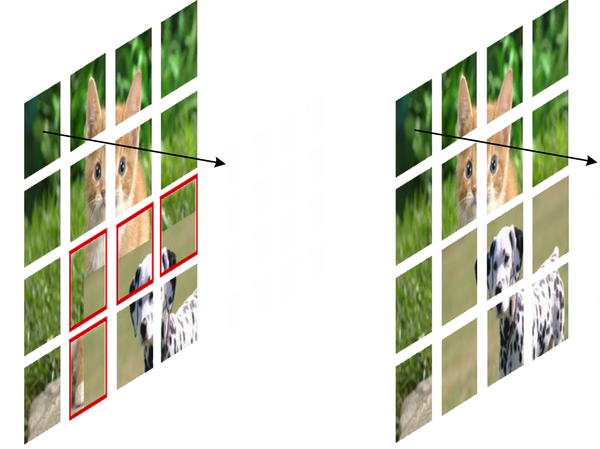$T_1=[t^1_1,...,t^N_1], T_2=[t^1_2,...,t^N_2]$ 。接下来通过一个mask $M$ 得到一个新的sequence of tokens：

$$\begin{equation} \hat{T}=T_1\odot M + T_2 \odot (1-M), \end{equation} \tag{21.4}$$

$$\begin{equation} \hat{Y}=Y_1\odot M + Y_2 \odot (1-M). \end{equation} \tag{21.5}$$

class token的标签可以写为：

$$\begin{equation} \hat{y^{cls}}= \bar{M} y_1^{cls} + (1 - \bar{M}) y_2^{cls}, \end{equation} \tag{21.6}$$

Experiments：

weight decay rate 0.05
warm up epochs 5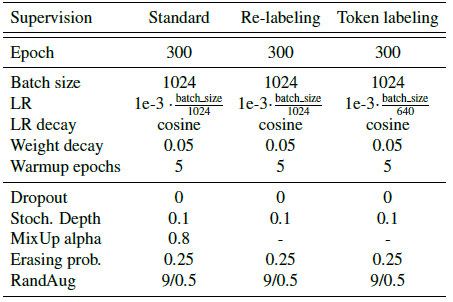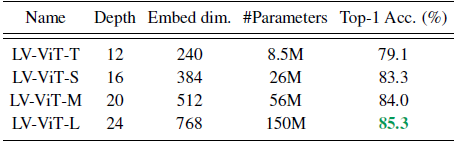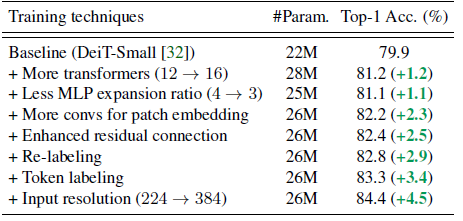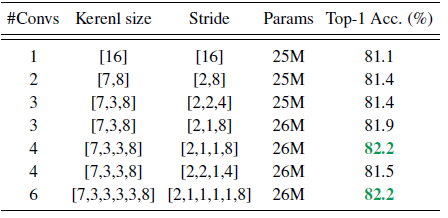$$Y=2X+0.3=X+F_1(X) \$$

$$Y=2X+0.3=X+F_2(X)/2 \$$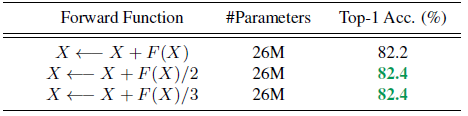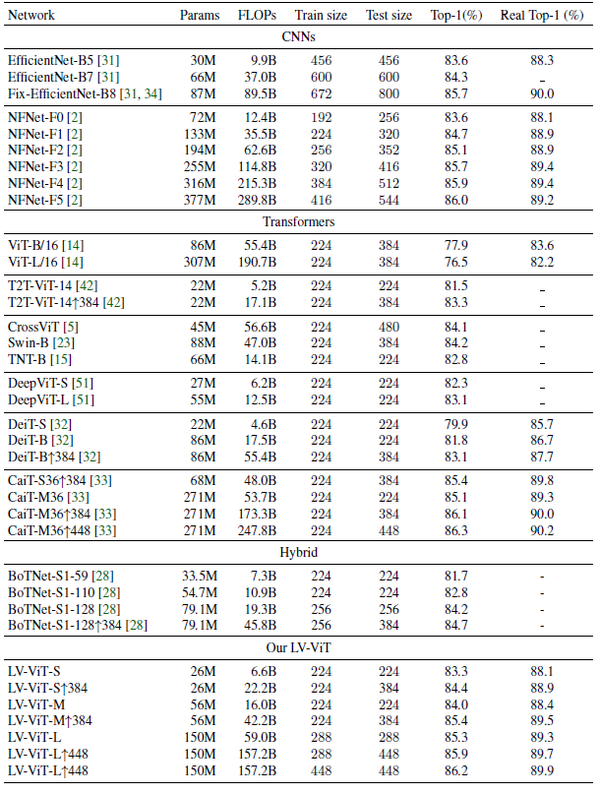## 22 通过抑制过度平滑来改进视觉Transformer训练

Over-smoothing

https://arxiv.org/pdf/2104.12753.pdf​arxiv.org

• 22.1 原理分析：

$$\mathrm{CosSim}(\bm{h}) = \frac{1}{n(n-1)} \sum_{i\neq j} \frac{h_i^\top h_j}{\parallel h_i\parallel \parallel h_j\parallel}, \tag{22.1}$$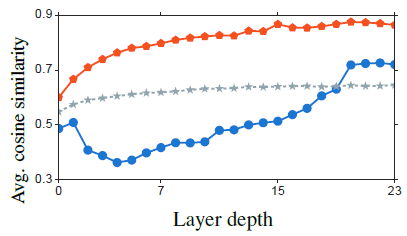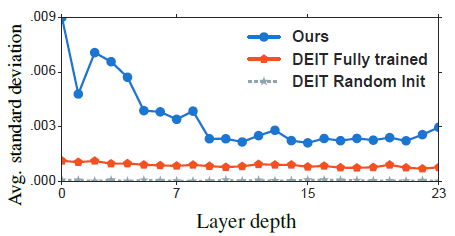1 添加相似度罚项：

$$\ell{\textit{cos}} = \frac{1}{n(n-1)} \sum{i\neq j} \frac{h_i^\top h_j}{\parallel h_i\parallel \parallel h_j \parallel}. \tag{22.2}$$

2 深层特征与浅层对应特征接近 (+ Patch Contrastive Loss)：

$$\ell{\textit{cons}} = - \frac{1}{n} \sum{i=1}^n \log \frac{\exp(e_i^\top h_i)}{\exp(e_i^\top h_i) + \exp(ei^\top (\sum{j=1}^n h_i / n))}, \tag{22.3}$$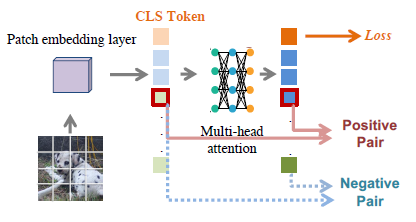3 每个Patch都有监督信息 (+ Patch Mixing Loss)：

Patch Mixing Loss定义为：

$$\ell\textit{token} = \frac{1}{n} \sum{i=1}^n \ell_\textit{ce}(g(h_i), y_i), \tag{22.4}$$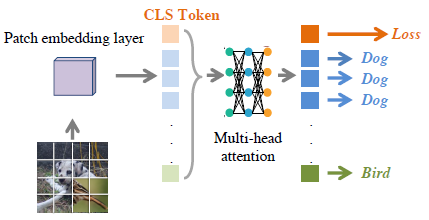Experiments：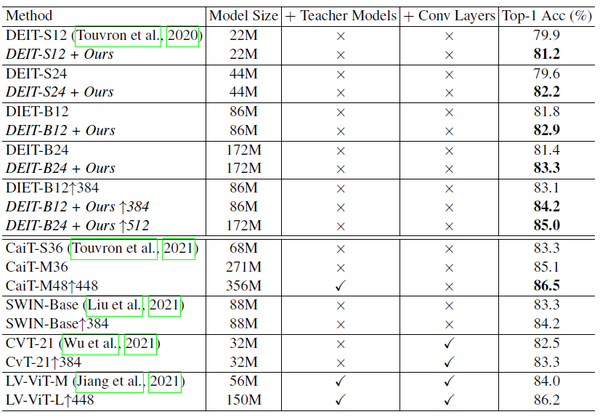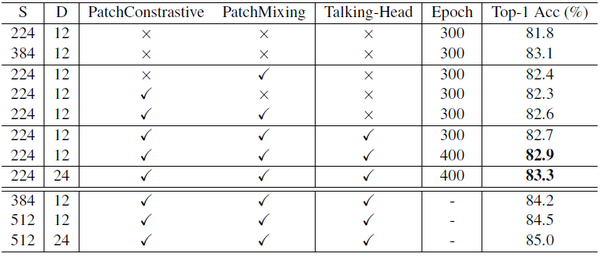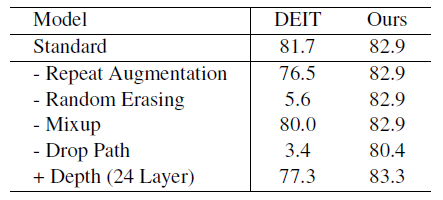Drop path rate是指Transformer的一个超参数，代表Dropout的比例。如下图19所示，添加了Patch Contrastive Loss 和 Patch Mixing Loss以后可以使用更大的drop path rate。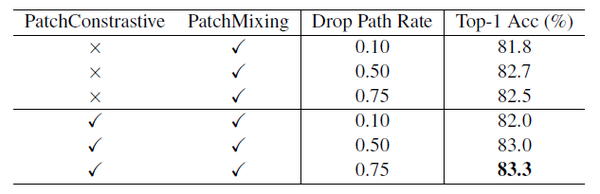## 总结：

•0
•0
•132收藏

• 13,409

关注
• 283

获赞
• 50

精选文章

• 从事AI视觉算法开发多年，主攻目标检测、图像分割方向

• 优质论文推荐

• 46
• 147
• 132
• 65
• 95